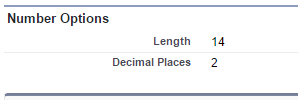## Getting length of integer part and fractional part in apex of a number type field salesforce

Sometimes we need to play with field (custom field or standard) properties in apex. If we need to find the integer part length in a “Number” type field, then there is no direct method in Describe Field Result (DescibeFieldResult) class. But we can find it using some other methods of DescribeFieldResult class.

Lets suppose we have a custom field of Number type with below properties.

“Test Number Field” (Test_Number_Field__c) Number(14, 2).Number type field options.

So here from the above custom field we want to fetch Length property from number options i.e. 14. But when we use getPrecision() method we will get 16, and when we use getScale() method we will get 2.

Output of the above apex code snippet will be :Apex Code Output.

So here we get the length of our Integer part in apex.

##### Conclusion

Integer Part Length = dfr.getPrecision() – dfr.getScale();

Fractional Part Length = dfr.getScale();

Total Length = dfr.getPrecision();

Finally if you need to do anything with the integer part length then grab the code from here and make it fast.

Happy Coding 🙂

This site uses Akismet to reduce spam. Learn how your comment data is processed.